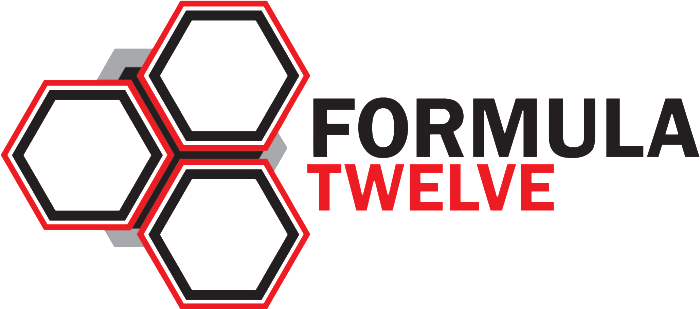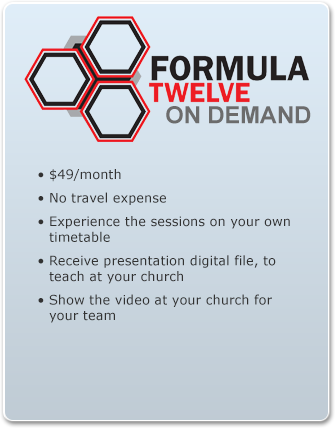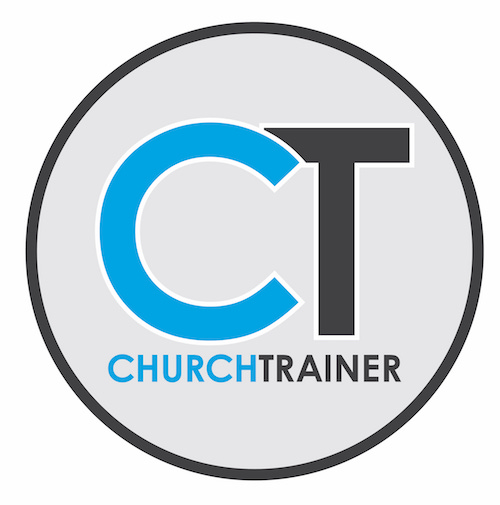Select PageFormula Twelve will lead you through monthly sessions (12) that cover proven strategies that will help your church grow. Each month you will uncover a new formula that is essential to leading your church to the next level!

Session Topics Include:

• Formula of Vision: The 26 Day Law
• Formula of Reaching People: The 80% Truth
• Formula of Communication: The 2+2+2 Strategy
• Formula of Life Giving Worship: The 10 Responses
• Formula of Events: The 10 Week Rule
• Formula of Impressing Guests: The 7×5 Law
• Formula of Connecting Guests: The 48 Hour Window
• Formula of Next Steps: The ¼ Goal
• Formula of Community: The 1 Focus Principle
• Formula of Care: The 7:100 Ratio
• Formula of Measurement: Attendance/Follow-Up Connection